# 🏕 Annotations, Markers, Logos, etc.

## Annotations

Static annotations can be added to the map via Maps.add_annotation().

• If a dataset has been plotted, you can mark any datapoint via its ID, e.g. using ID=...

• To add a marker at an arbitrary position, use xy=(...)

• By default, the coordinates are assumed to be provided in the plot-crs

• You can specify arbitrary coordinates via xy_crs=...

• Additional arguments are passed to matplotlib.pyplot.annotate and matplotlib.pyplot.text

• This gives a lot of flexibility to style the annotations!

To dynamically add annotations if you click on the map, checkout the associated pre-defined callback m.cb.click.attach.annotate() (or m.cb.pick.attach.annotate())

 Maps.add_annotation Add an annotation to the plot.

Interactively add annotations and re-position/re-style existing annotations

The Companion Widget provides some capabilities to quickly put annotations on a map via the Add Annotations tab.

Starting with EOmaps v7.0 it is also possible to interactively edit existing annotations by activating the Edit Annotations button.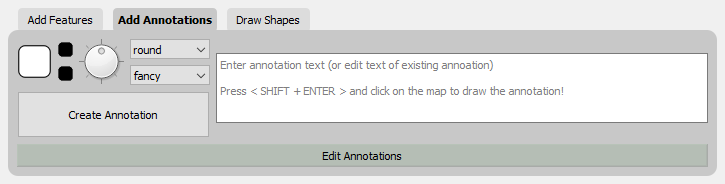from eomaps import Maps import numpy as np x, y = np.mgrid[-45:45, 20:60] m = Maps(crs=4326) m.set_data(x+y, x, y) m.add_feature.preset.coastline(ec="k", lw=.75) m.plot_map() # annotate any point in the dataset via the data-index m.add_annotation(ID=345) # annotate an arbitrary position (in the plot-crs) m.add_annotation( xy=(20,25), text="A formula:\n $z=\sqrt{x^2+y^2}$", fontweight="bold", bbox=dict(fc=".6", ec="none", pad=0.2)) # annotate coordinates defined in arbitrary crs m.add_annotation( xy=(2873921, 6527868), xy_crs=3857, xytext=(5,5), text="A location defined \nin epsg 3857", fontsize=8, rotation=-45, bbox=dict(fc="skyblue", ec="k", ls="--", pad=0.2)) # functions can be used for more complex text def text(m, ID, val, pos, ind): return f"lon={pos}\nlat={pos}" props = dict(xy=(-1.5, 38.45), text=text, arrowprops=dict(arrowstyle="-|>", fc="fuchsia", mutation_scale=15)) m.add_annotation(**props, xytext=(20, 20), color="darkred") m.add_annotation(**props, xytext=(-60, 20), color="purple") m.add_annotation(**props, xytext=(-60, -40), color="dodgerblue") m.add_annotation(**props, xytext=(20, -40), color="olive") # multiple annotations can be added in one go (xy=([...], [...]) also works!) m.add_annotation(ID=[2500, 2700, 2900], text=lambda ID, **kwargs: str(ID), color="w", fontweight="bold", rotation=90, arrowprops=dict(width=5, fc="b", ec="orange", lw=2), bbox=dict(boxstyle="round, rounding_size=.8, pad=.5", fc="b", ec="orange", lw=2)) m.add_annotation(ID=803, xytext=(-80,60), bbox=dict(ec="r", fc="gold", lw=3), arrowprops=dict( arrowstyle="fancy", relpos=(.48,-.2), mutation_scale=40, fc="r", connectionstyle="angle3, angleA=90, angleB=-25"))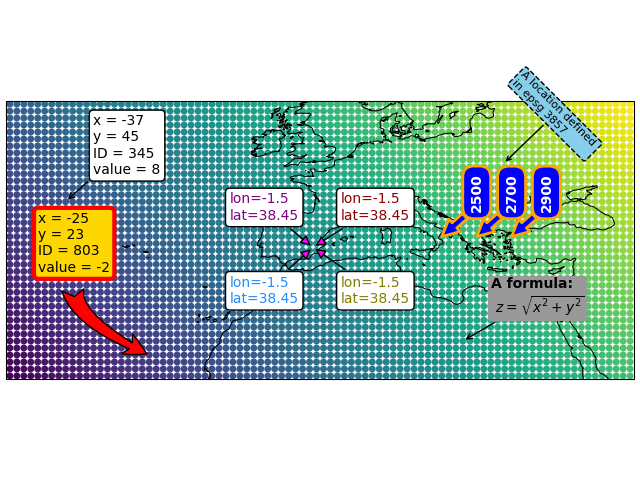## Markers

Static markers can be added to the map via Maps.add_marker().

• If a dataset has been plotted, you can mark any datapoint via its ID, e.g. ID=...

• To add a marker at an arbitrary position, use xy=(...)

• By default, the coordinates are assumed to be provided in the plot-crs

• You can specify arbitrary coordinates via xy_crs=...

• The radius is defined via radius=...

• By default, the radius is assumed to be provided in the plot-crs

• You can specify the radius in an arbitrary crs via radius_crs=...

• The marker-shape is set via shape=...

• Possible arguments are "ellipses", "rectangles", "geod_circles"

• Additional keyword-arguments are passed to the matplotlib collections used to draw the shapes (e.g. “facecolor”, “edgecolor”, “linewidth”, “alpha”, etc.)

• Multiple markers can be added in one go by using lists for xy, radius, etc.

To dynamically add markers if you click on the map, checkout the associated pre-defined callback m.cb.click.attach.mark() (or m.cb.pick.attach.mark())

 Maps.add_marker Add a marker to the plot.
 from eomaps import Maps m = Maps(crs=4326) m.add_feature.preset.coastline() # ----- SINGLE MARKERS # by default, MARKER DIMENSIONS are defined in units of the plot-crs! m.add_marker(xy=(0, 0), radius=20, shape="rectangles", fc="y", ec="r", ls=":", lw=2) m.add_marker(xy=(0, 0), radius=10, shape="ellipses", fc="darkorange", ec="r", ls=":", lw=2) # MARKER DIMENSIONS can be specified in any CRS! m.add_marker(xy=(12000000, 0), xy_crs=3857, radius=5000000, radius_crs=3857, fc=(.5, .5, 0, .4), ec="r", lw=3, n=100) # GEODETIC CIRCLES with radius defined in meters m.add_marker(xy=(-135, 35), radius=3000000, shape="geod_circles", fc="none", ec="r", hatch="///", lw=2, n=100) # ----- MULTIPLE MARKERS x = [-80, -40, 40, 80] # x-coordinates of the markers fc = ["r", "g", "b", "c"] # the colors of the markers # N markers with the same radius m.add_marker(xy=(x, [-60]*4), radius=10, fc=fc) # N markers with different radius and properties m.add_marker(xy=(x, *4), radius=[15, 10, 5, 2], fc=fc, ec=["none", "r", "g", "b"], alpha=[1, .5, 1, .5]) # N markers with different widths and heights radius = ([15, 10, 5, 15], [5, 15, 15, 2]) m.add_marker(xy=(x, *4), radius=radius, fc=fc)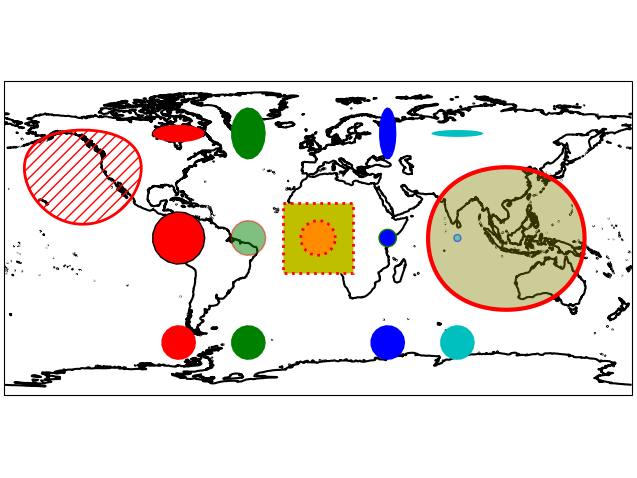## Lines

Lines can be added to a map with Maps.add_line().

• A line is defined by a list of anchor-points and a connection-method

• The coordinates of the anchor-points can be provided in any crs

• Possible connection-methods are:

• connect="geod": connect points via geodesic lines (the default)

• use n=10 to calculate 10 intermediate points between each anchor-point

• or use del_s=1000 to calculate intermediate points (approximately) every 1000 meters

• connect="straight": connect points via straight lines

• connect="straight_crs": connect points with reprojected lines that are straight in a given projection

• use n=10 to calculate 10 (equally-spaced) intermediate points between each anchor-point

• Additional keyword-arguments are passed to matplotlib.pyplot.plot

• This gives a lot of flexibility to style the lines!

 Maps.add_line Draw a line by connecting a set of anchor-points.
 from eomaps import Maps import matplotlib.patheffects as path_effects m = Maps(Maps.CRS.Sinusoidal(), figsize=(8, 4)) m.add_feature.preset.ocean() p0 = [(-100,10), (34, -56), (125, 57)] p1 = [(-120,50), (-42, 63), (45, 57)] p2 = [(-20,-45), (-20, 45), (45, 45), (45, -20), (-20,-45)] m.add_line(p0, connect="geod", del_s=100000, lw=0.5, c="k", mark_points="rs", marker=".", markevery=10) m.add_line(p1, connect="straight", c="b", ls="--", mark_points=dict(fc="y", ec="k", lw=.5)) m.add_line(p2, connect="straight_crs", c="r", n=5, lw=0.25, ms=5, path_effects=[ path_effects.withStroke(linewidth=3, foreground="gold"), path_effects.TickedStroke(angle=90, linewidth=1, length=0.5)])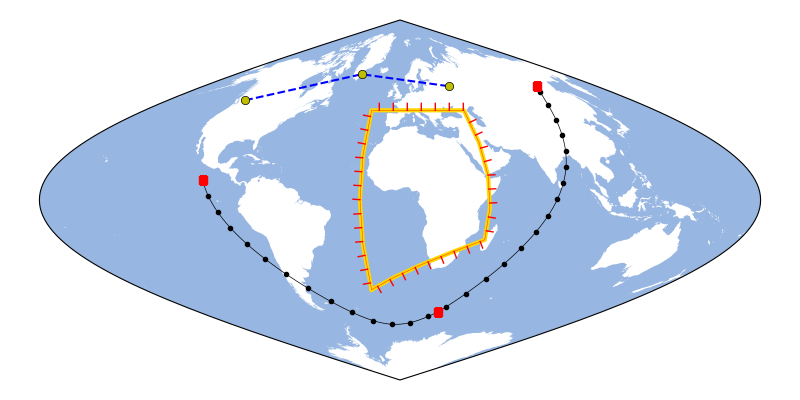## Rectangular areas

To indicate rectangular areas in any given crs, simply use Maps.indicate_extent():

 Maps.indicate_extent Indicate a rectangular extent in a given crs on the map.
 from eomaps import Maps m = Maps(crs=3035) m.add_feature.preset.coastline(ec="k") # indicate a lon/lat rectangle m.indicate_extent(-20, 35, 40, 50, hatch="//", fc="none", ec="r") # indicate some rectangles in epsg:3035 hatches = ["*", "xxxx", "...."] colors = ["yellow", "r", "darkblue"] for i, h, c in zip(range(3), hatches, colors): pos0 = (2e6 + i*2e6, 7e6, 3.5e6 + i*2e6, 9e6) pos1 = (2e6 + i*2e6, 7e6 + 3e6, 3.5e6 + i*2e6, 9e6 + 3e6) m.indicate_extent(*pos0, crs=3857, hatch=h, lw=0.25, ec=c) m.indicate_extent(*pos1, crs=3857, hatch=h, lw=0.25, ec=c) # indicate a rectangle in European Equi7Grid projection m.indicate_extent(1000000, 1000000, 4800000, 4800000, crs=Maps.CRS.Equi7_EU, fc="g", alpha=0.5, ec="k")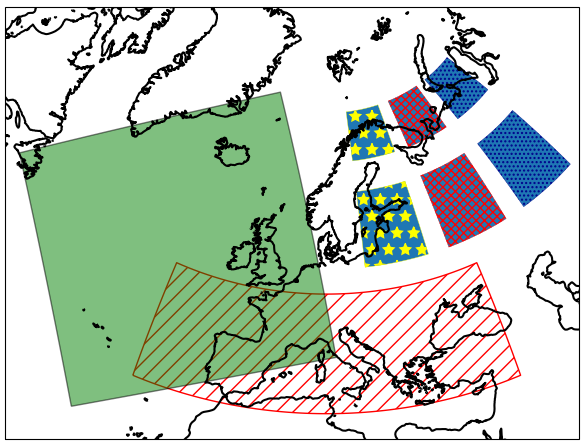## Logos

To add a logo (or basically any image file .png, .jpeg etc.) to the map, you can use Maps.add_logo().

Logos can be re-positioned and re-sized with the 🏗️ Layout Editor!

• To fix the relative position of the logo with respect to the map-axis, use fix_position=True

 from eomaps import Maps m = Maps() m.add_feature.preset.coastline() m.add_logo(position="ul", size=.15) m.add_logo(position="ur", size=.15) # notice that the bottom logos maintain their relative position on resize/zoom events! # (and also that they can NOT be moved with the layout-editor) m.add_logo(position="lr", size=.3, pad=(0.1,0.05), fix_position=True) m.add_logo(position="ll", size=.4, fix_position=True)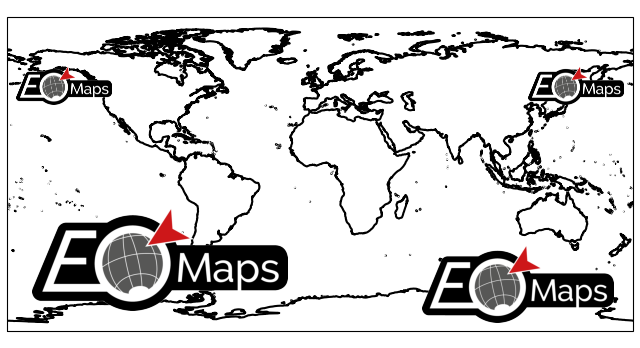Maps.add_logo Add a small image (png, jpeg etc.) to the map.